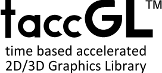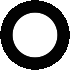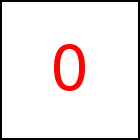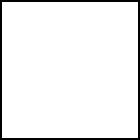# External 3D Models - taccGL™ Tutorial

In section Basic 3D Models we have already explained how to show and animate an external 3D Model. This section and its subsections introduces various features of taccgl™ w.r.t. 3D models such as how to modify, align, color, and texture models. In addition there are subsections explaining how to best export 3D models from modelling porgrams, how to put them on a web server, and how to more efficiently download them.

## Selecting Parts of a Scene

almost empty div element with id="ex20"

The examples shown so far used the scene method to display a 3D model of a complete scene. In addition there is the objs to pick some of the objects from the scene for display.

In the first two examples below objs gets the name of the object to display. Actually objs takes a regular expression as parameter and includes all objects of the 3D model that match. So it is, as in the third example below, possible to select multiple objects, separating the names with "|".

This technique has various applications: It is possible to have various objects for use in differenct places in one model, thereby reducing the number of model files that need to be maintained and downloaded. The taccgldoc.obj for example contains various objects, e.g. a Sphere, a Cylinder, and a box. You can also design a 3D model with exchangable parts, e.g. a cupboard with either and open or a closed door and then select with objs either to include the close or the open door plus the rest of the cupboard.

almost empty div element with id="ex40"

The next example shows another application which is to animate various parts of a model differently. Basically the idea is to have multiple taccgl™ transitions, each using the same 3D model at the same HTML element, but selecting different parts of the model and having different animations.

 var to=taccgl. objFile().read('/objtest/taccgldoc.obj',false); to.mtl.ambientAdjust(0.4); taccgl.actor("ex40",to.objs("Sphere|Torus")) .modFit() .rotateMiddle(0.7,0.7,0) .dur(3).start(); taccgl.actor("ex40",to.objs("Cylinder|Icosphere")) .modFit() .rotateMiddle(-0.7,-0.7,1) .dur(3).start(); RUN

## Scaling and Aligning 3D Models with HTML Elements (modFit)

A 3D scene displayed with taccgl™ must either already have the right size or it can be resized and aligned with the placehodler HTML elements. See the box below for the rules of manual alignment and the rest of the section using automatic alignment.

When showing a 3D Model using a placeholder HTML element and not using any aligment or scaling methods, the (0,0,0) point of the 3D model is mapped to the top left corner of the HTML element, the (1,0,0) point to the top right corner, and the (0,1,0) point to the bottom left corner. taccgl™ assumes a depth d of the object identical to the height of the HTML elements. The point (0,0,-1) is mapped to a point d pixels "in front" of the top left corner of the HTML element.

The 3D model can exceed the unit cube, but then probably will exceed the placeholder element. If the placeholder element is not quadratic the 3D models aspect ration will change and the model look distorted.

Both examples below do not use automatic scaling. The first one fits well with the placeholder HTML since the 3D model has the size of a unit cube. You will notice, however, that the model is not cubic which comes from the fact that the model is stretched according to the underlying placeholder HTML, which is not quadratic. The second example does not fit at all with the placeholder, since the 3D model is much bigger than the unit cube

 var to=taccgl. objFile().read('/tutorial/obj/secondExample.obj',false); taccgl.a("ex60",to.scene()) .dur(3).start(); RUN var to=taccgl. objFile().read('/objtest/taccgldoc.obj',false); taccgl.a("ex60",to.objs("Cube")) .dur(3).start(); RUN
almost empty div element with id="ex60"

Automatic scaling and alignment is done using modfit which may or may not have parameters. If none of the parameters is given, then the 3D model is aligned and stretched to exactly fit the HTML element. More precisely the HTML element is extended to a box, whereby the HTML element is the front plane of the box. The box has a depth d, which is per default identical to the height of the HTML element.

The following 2 examples extend the previous 2 examples by using modfit without parameters. You will notice, that modfit does not change the first example but the second example is scaled and aligned to fit into the box of the HTML element.

 var to=taccgl. objFile().read('/tutorial/obj/secondExample.obj',false); taccgl.a("ex80",to.scene()) . modFit() .dur(3).start(); RUN var to=taccgl. objFile().read('/objtest/taccgldoc.obj',false); taccgl.a("ex80",to.objs("Cube")) . modFit() .dur(3).start(); RUN
almost empty div element with id="ex80"

The following examples use modfit with an alignment argument. If used, the 3D model is resized to fit the HTML element while keeping its aspect ratio. The alignment parameter is a 3 letter string, the first letter standing for alignment in x-direction, the second for y-direction, etc. So for example "ltf" means left-top-front and "mmm" middle-middle-middle alignment. While viewing the following examples it is useful to show the box as well box,

 var to=taccgl. objFile().read('/objtest/taccgldoc.obj',false); taccgl.a("ex80",to.objs("Sphere")) .modFit("ltf") .dur(3).start(); RUN var to=taccgl. objFile().read('/objtest/taccgldoc.obj',false); taccgl.a("ex80",to.objs("Sphere")) .modFit("mmm") .dur(3).start(); RUN var to=taccgl. objFile().read('/objtest/taccgldoc.obj',false); taccgl.a("ex80",to.objs(".*")) .modFit("mmm") .dur(3).start(); RUN var to=taccgl. objFile().read('/objtest/roundcube/roundcube1.obj',false); taccgl.a("ex80",to.scene()) .modFit("mmm") .dur(3).start(); RUN var to=taccgl. objFile().read('/tutorial/obj/secondExample.obj',false); taccgl.a("ex80",to.scene()) .modFit("mmm" ) .dur(3).start(); RUN var to=taccgl. objFile().read('/objtest/taccgldoc.obj',false); taccgl.a("ex80",to.objs("Sphere")) .modFit("mmF") .dur(3).start(); RUN var to=taccgl. objFile().read('/objtest/taccgldoc.obj',false); taccgl.a("ex80",to.objs("Sphere")) .modFit("mTm") .dur(3).start(); RUN

There are many more alignment option described in the manual: See modfit. For example the alignments used in the last 2 examples above: "mmF" means middle-middle-in-front-of-the-box and "mTm" middle-on-Top-of-the-box-middle.

Note that the second example above rotates around the center of the placeholder HTML element.

WebGL™ is a trademark of the Khronos Group Inc.

# Tutorial

taccgl™ - HTML5 Canvas 3D Animation Library